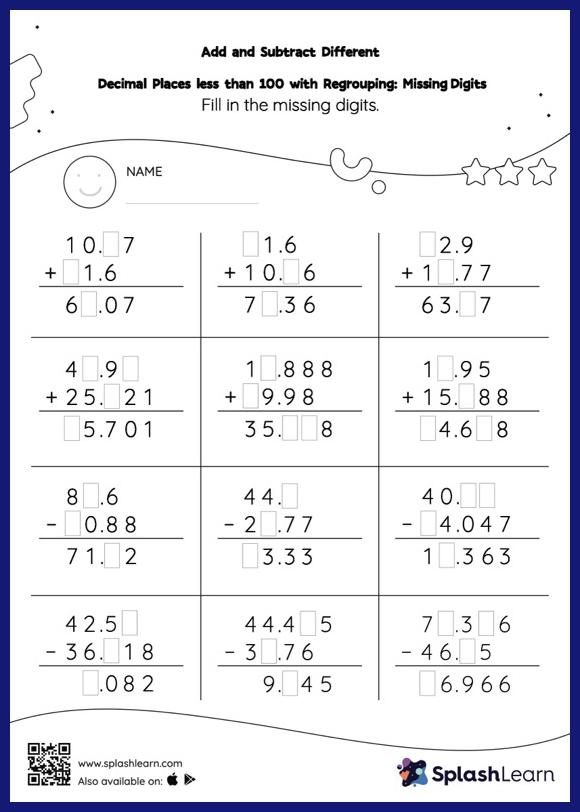# Add and Subtract Different Decimal Places less than 100 with Regrouping: Missing Digits Worksheet

Home > Add and Subtract Different Decimal Places less than 100 with Regrouping: Missing DigitsStudents find the missing number while adding or subtracting decimals by using the relationship between addition and subtraction. To reach the answer, they also use the relationship between ones, tenths, hundredths, thousandths, etc., To regroup the numbers in add and subtract different decimal places less than 100 with regrouping worksheet. In this worksheet, students practice solving problems using the column method. This method is especially helpful with problems involving bigger multi-digit numbers as the format provides an easy structure to follow.